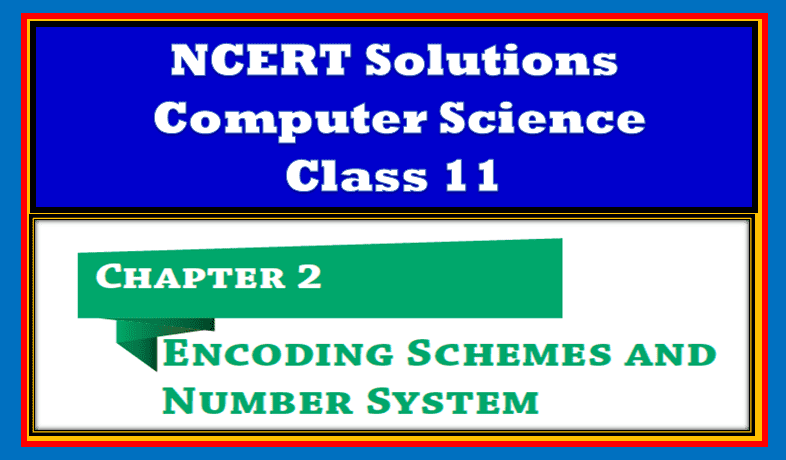In this article, I will provide Chapter 2 Encoding Scheme and Number System Class 11 NCERT Solutions. So let’s start1

## Chapter 2 Encoding Scheme and Number System Class 11

Let’s start the solution of the encoding scheme and number system class 11 with basic questions.

1. Write base values of binary, octal and hexadecimal number system.
• Binary – 2
• Octal – 8
2. Give full form of ASCII and ISCII.
• ASCII – Americal Strandard Code for Information Interchange
• ISCII – Indian Script Code for Information Interchange
3. Try the following conversions:

(IV) Do yourself.

(VI) Do yourself.

1. Do the following conversions from decimal number to other number systems.

(ii) Do yourself.

(iv) Do yourself.

(vi) Do yourself

5. Express the following octal numbers into their equivalent decimal numbers.
(i) 145 (ii) 6760 (iii) 455 – Do yourself.

6. Express the following decimal numbers into hexadecimal numbers.
(i) 548 (ii) 4052 (iii) 58 – Do yourself.

1. Express the following hexadecimal numbers into equivalent decimal numbers.

(ii) and (iii) do by yourself.

1. Convert the following binary numbers into octal and hexadecimal numbers.
Whenever you are going to convert binary to hexadecimal follow the shortcut method.

(ii) 110110101 (iii) 1010100 (iv) 1010.1001 – Do yourself.

1. Write binary equivalent of the following octal numbers.

(ii) 5610 (iii) 742 (iv) 65.203 – Do yourslef.

If you are looking for a calculator to check the conversion follow this link: Rapid tables

1. Write binary representation of the following hexadecimal numbers: (i) 4026 (ii) BCA1 (iii) 98E (iv) 132.45 – Do yourself.
2. How does computer understand the following text? (hint: 7 bit ASCII code).

(i) HOTS

• ASCII value of H is 72 and its equivalent 7-bit binary code = 1001000
• ASCII value of O is 79 and its equivalent 7-bit binary code = 1001111
• ASCII value of S is 83 and its equivalent 7-bit binary code = 1010011
• ASCII value of T is 84 and its equivalent 7-bit binary code = 1010100

(ii) Main

• ASCII value of M is 77 and its equivalent 7-bit binary code = 1001101
• ASCII value of a is 97 and its equivalent 7-bit binary code = 1100001
• ASCII value of i is 105 and its equivalent 7-bit binary code = 1101001
• ASCII value of n is 110 and its equivalent 7-bit binary code = 1101110

(iii) CaSe – do yourself.

1. The hexadecimal number system uses 16 literals (0 – 9, A– F). Write down its base value. – Do yourself
2. Let X be a number system having B symbols only. Write down the base value of this number system. – Do yourself
3. Write the equivalent hexadecimal and binary values for each character of the phrase given below. – ‘‘ हम सब एक”
• ह – 0939
• म – 092E
• स – 0938
• ब – 092C
• ए – 090F
• क – 0915
1. What is the advantage of preparing a digital content in Indian language using UNICODE font?
• It allows to incorporate all the characters of every written language.
• It provides a unique number for every character
• Every character represented thorugh unicode is compatible for various devices like mobile, tables, computer and os like Linux, Windows, Android etc.
1. Explore and list the steps required to type in an Indian language using UNICODE.

Step 1: Type the instruction in the Indian language
Step 2: Each character has its own hexadecimal code that the machine will read that code.
Step 3: The machine will then convert that UNICODE to binary code and do the task according to the instruction given

1. Encode the word ‘COMPUTER’ using ASCII and convert the encode value into binary values. – Do yourself